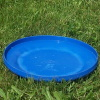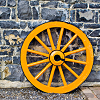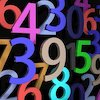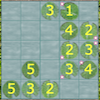# Resources tagged with: Interactivities

Filter by: Content type:
Age range:
Challenge level:

### There are 94 results

Broad Topics > Physical and Digital Manipulatives > Interactivities### Two's Company

##### Age 11 to 14Challenge Level

Seven balls are shaken. You win if the two blue balls end up touching. What is the probability of winning?### Which Spinners?

##### Age 14 to 18Challenge Level

Can you work out which spinners were used to generate the frequency charts?### Flippin' Discs

##### Age 11 to 14Challenge Level

Discs are flipped in the air. You win if all the faces show the same colour. What is the probability of winning?### Interactive Spinners

##### Age 11 to 14Challenge Level

This interactivity invites you to make conjectures and explore probabilities of outcomes related to two independent events.### Cosy Corner

##### Age 11 to 14Challenge Level

Six balls are shaken. You win if at least one red ball ends in a corner. What is the probability of winning?### Red or Black Spinner

##### Age 3 to 16Challenge Level

A simple spinner that is equally likely to land on Red or Black. Useful if tossing a coin, dropping it, and rummaging about on the floor have lost their appeal. Needs a modern browser; if IE then at. . . .##### Age 14 to 16Challenge Level

A counter is placed in the bottom right hand corner of a grid. You toss a coin and move the star according to the following rules: ... What is the probability that you end up in the top left-hand. . . .### Excel Interactive Resource: Fraction Multiplication

##### Age 11 to 16Challenge Level

Use Excel to explore multiplication of fractions.### Excel Interactive Resource: Equivalent Fraction Bars

##### Age 11 to 16Challenge Level

A simple file for the Interactive whiteboard or PC screen, demonstrating equivalent fractions.### Excel Interactive Resource: Interactive Division

##### Age 11 to 16Challenge Level

Use an Excel to investigate division. Explore the relationships between the process elements using an interactive spreadsheet.### Cushion Ball Interactivity

##### Age 14 to 16Challenge Level

The interactive diagram has two labelled points, A and B. It is designed to be used with the problem "Cushion Ball"### Excel Interactive Resource: Number Grid Functions

##### Age 11 to 16Challenge Level

Use Excel to investigate the effect of translations around a number grid.### Excel Interactive Resource: the up and Down Game

##### Age 11 to 16Challenge Level

Use an interactive Excel spreadsheet to explore number in this exciting game!### Excel Interactive Resource: Multiples Chain

##### Age 11 to 16Challenge Level

Use an interactive Excel spreadsheet to investigate factors and multiples.### Excel Interactive Resource: Long Multiplication

##### Age 11 to 16Challenge Level

Use an Excel spreadsheet to explore long multiplication.### Excel Interactive Resource: Fraction Addition & Fraction Subtraction

##### Age 11 to 16Challenge Level

Use Excel to practise adding and subtracting fractions.### Excel Interactive Resource: Haitch

##### Age 11 to 16Challenge Level

An Excel spreadsheet with an investigation.### Magic W

##### Age 14 to 16Challenge Level

Find all the ways of placing the numbers 1 to 9 on a W shape, with 3 numbers on each leg, so that each set of 3 numbers has the same total.### Olympic Magic

##### Age 14 to 16Challenge Level

in how many ways can you place the numbers 1, 2, 3 … 9 in the nine regions of the Olympic Emblem (5 overlapping circles) so that the amount in each ring is the same?### Instant Insanity

##### Age 11 to 18Challenge Level

Given the nets of 4 cubes with the faces coloured in 4 colours, build a tower so that on each vertical wall no colour is repeated, that is all 4 colours appear.### Drips

##### Age 7 to 14Challenge Level

An animation that helps you understand the game of Nim.### Changing Places

##### Age 14 to 16Challenge Level

Place a red counter in the top left corner of a 4x4 array, which is covered by 14 other smaller counters, leaving a gap in the bottom right hand corner (HOME). What is the smallest number of moves. . . .### Tilted Squares

##### Age 11 to 14Challenge Level

It's easy to work out the areas of most squares that we meet, but what if they were tilted?### Bow Tie

##### Age 11 to 14Challenge Level

Show how this pentagonal tile can be used to tile the plane and describe the transformations which map this pentagon to its images in the tiling.### Partitioning Revisited

##### Age 11 to 14Challenge Level

We can show that (x + 1)² = x² + 2x + 1 by considering the area of an (x + 1) by (x + 1) square. Show in a similar way that (x + 2)² = x² + 4x + 4### The Bridges of Konigsberg

##### Age 11 to 18Challenge Level

Investigate how networks can be used to solve a problem for the 18th Century inhabitants of Konigsberg.### Guesswork

##### Age 14 to 16Challenge Level

Ask a friend to choose a number between 1 and 63. By identifying which of the six cards contains the number they are thinking of it is easy to tell them what the number is.### Rollin' Rollin' Rollin'

##### Age 11 to 14Challenge Level

Two circles of equal radius touch at P. One circle is fixed whilst the other moves, rolling without slipping, all the way round. How many times does the moving coin revolve before returning to P?### Rolling Around

##### Age 11 to 14Challenge Level

A circle rolls around the outside edge of a square so that its circumference always touches the edge of the square. Can you describe the locus of the centre of the circle?### Number Pyramids

##### Age 11 to 14Challenge Level

Try entering different sets of numbers in the number pyramids. How does the total at the top change?### Sliding Puzzle

##### Age 11 to 16Challenge Level

The aim of the game is to slide the green square from the top right hand corner to the bottom left hand corner in the least number of moves.### Proof Sorter - Quadratic Equation

##### Age 14 to 18Challenge Level

This is an interactivity in which you have to sort the steps in the completion of the square into the correct order to prove the formula for the solutions of quadratic equations.### Overlap

##### Age 11 to 14Challenge Level

A red square and a blue square overlap so that the corner of the red square rests on the centre of the blue square. Show that, whatever the orientation of the red square, it covers a quarter of the. . . .### Icosian Game

##### Age 11 to 14Challenge Level

This problem is about investigating whether it is possible to start at one vertex of a platonic solid and visit every other vertex once only returning to the vertex you started at.### Picturing Triangular Numbers

##### Age 11 to 14Challenge Level

Triangular numbers can be represented by a triangular array of squares. What do you notice about the sum of identical triangle numbers?### Stars

##### Age 11 to 14Challenge Level

Can you work out what step size to take to ensure you visit all the dots on the circle?### More Number Pyramids

##### Age 11 to 14Challenge Level

When number pyramids have a sequence on the bottom layer, some interesting patterns emerge...### Countdown

##### Age 7 to 14Challenge Level

Here is a chance to play a version of the classic Countdown Game.### Latin Lilies

##### Age 7 to 14Challenge Level

In this game you are challenged to gain more columns of lily pads than your opponent.### Factors and Multiples Game

##### Age 7 to 16Challenge Level

A game in which players take it in turns to choose a number. Can you block your opponent?### Have You Got It?

##### Age 11 to 14Challenge Level

Can you explain the strategy for winning this game with any target?### Estimating Angles

##### Age 7 to 14Challenge Level

How good are you at estimating angles?### Poly Plug Rectangles

##### Age 5 to 14Challenge Level

The computer has made a rectangle and will tell you the number of spots it uses in total. Can you find out where the rectangle is?### Spinners Environment

##### Age 5 to 18Challenge Level

A tool for generating random integers.### Countdown Fractions

##### Age 11 to 16Challenge Level

Here is a chance to play a fractions version of the classic Countdown Game.### Mixed up Mixture

##### Age 14 to 16Challenge Level

Can you fill in the mixed up numbers in this dilution calculation?### An Unhappy End

##### Age 11 to 14Challenge Level

Two engines, at opposite ends of a single track railway line, set off towards one another just as a fly, sitting on the front of one of the engines, sets off flying along the railway line...### Investigating the Dilution Series

##### Age 14 to 16Challenge Level

Which dilutions can you make using only 10ml pipettes?### A Question of Scale

##### Age 14 to 16Challenge Level

Use your skill and knowledge to place various scientific lengths in order of size. Can you judge the length of objects with sizes ranging from 1 Angstrom to 1 million km with no wrong attempts?### Interactive Number Patterns

##### Age 14 to 16Challenge Level

How good are you at finding the formula for a number pattern ?